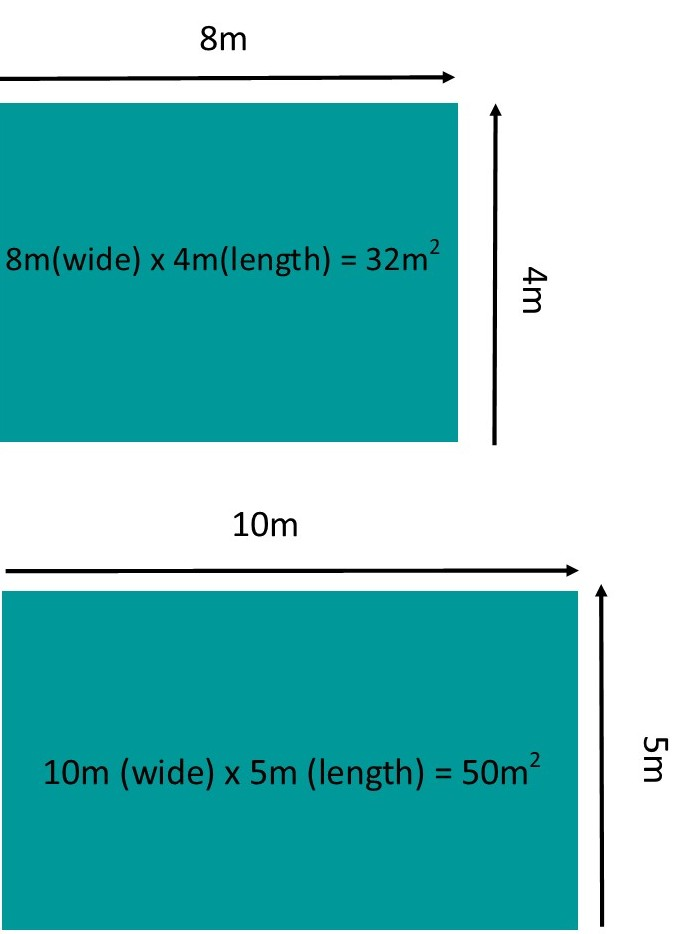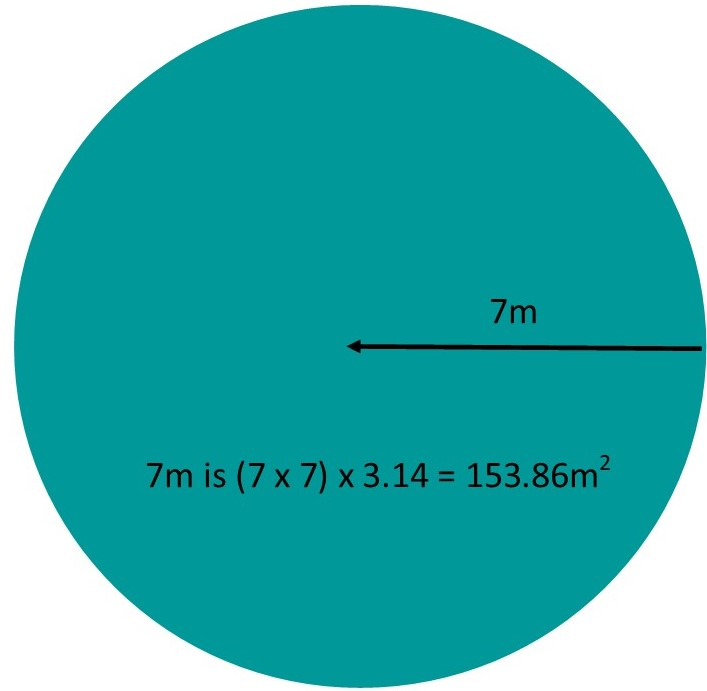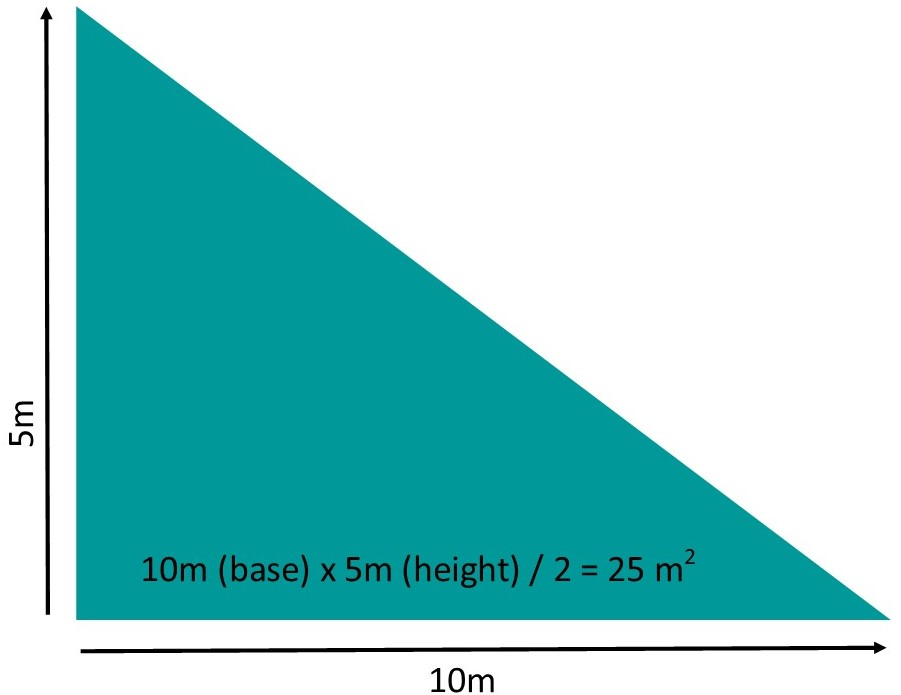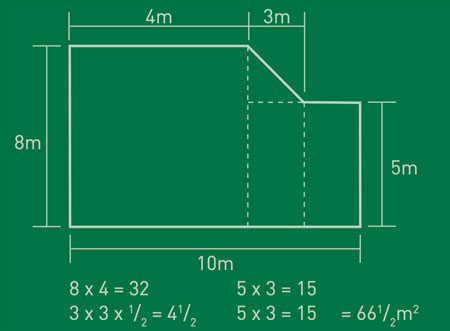# HOW TO MEASURE

Measuring your lawn is not that hard. There are just a few simple steps to follow and then you can ask for a final quote. ALWAYS remember to add 10% more to the final figure so you have extra for cutting and shaping.#### SQUARE AND RECTANGLE

If you’re lucky enough to have a square or rectangular lawn, measuring the area is simple. Measure the width and the length and multiply the two together. This gives you your area.

For example, a square – 8m (wide) x 4m (length) = 32m² + 10% = 35.2m²
For example, a rectangle – 10m (wide) x 5m (length) = 50m² + 10% = 55m²

Of course, not all lawns are that easy to measure. As people take more of an interest in the design of their gardens, irregular shapes are increasingly popular. If the shape is relatively simple and made-up of separate squares or rectangles, you can measure it by breaking it up.#### CIRCLE

For circular shaped lawns there is an easy formula. Measure the diameter which is the width of the circle, and the radius is half the width of the circle. To calculate the lawn size, measure the radius of the circle and multiple the (radius x radius) x 3.14.

For example, a circle – with a radius of 7m is (7 x 7) x 3.14 = 153.86m² + 10% = 169.25m²#### TRIANGLE

There are three different type of triangles your lawn may form, all are easy to measure. The area formula for any triangle is to multiply the base by the height and divide the total by two.

For example, if you have a right-angle triangle – 10m (base) x 5m (height) / 2 = 25 m² + 10% = 27.5m²#### IRREGULAR SHAPED LAWN

As a guide we recommend you firstly divide your irregularly shaped lawn into sections of regular shapes, for example, circle, square and triangle. These will be more manageable sections which are easier to measure. Find the individual area of each different shape and then add them together.

For example, a circle (3m radius) + rectangle lawn (4m wide x 7m length)
CIRCLE (3 x 3) x 3.14 = 28.26m²
RECTANGLE 4m (wide) x 7m (length) = 28m²
TOTAL AREA – 56.26m² + 10% = 61.89m²